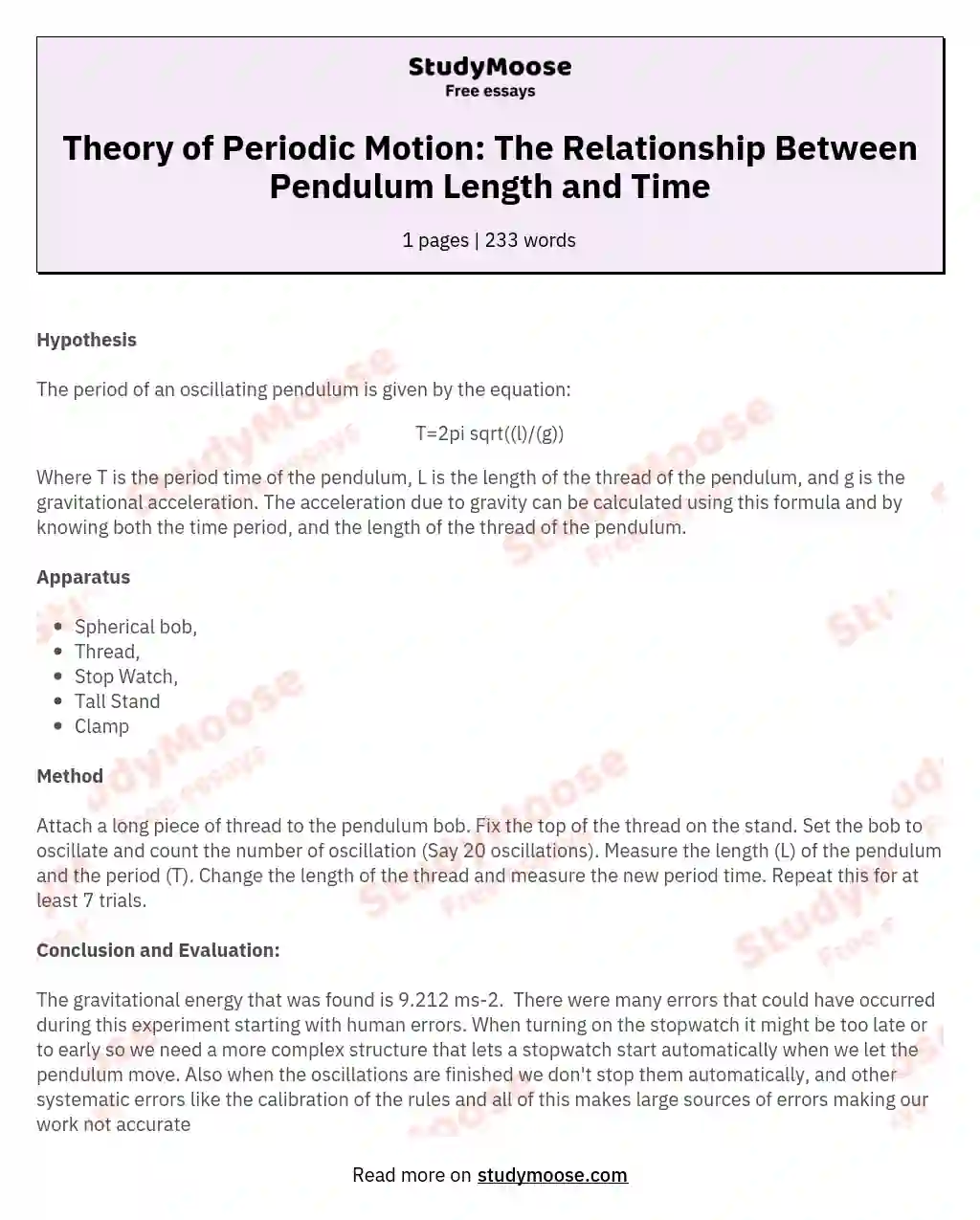# Theory of Periodic Motion: The Relationship Between Pendulum Length and Time

#### Hypothesis

The period of an oscillating pendulum is given by the equation:

T=2pi sqrt((l)/(g))

Where T is the period time of the pendulum, L is the length of the thread of the pendulum, and g is the gravitational acceleration.

The acceleration due to gravity can be calculated using this formula and by knowing both the time period, and the length of the thread of the pendulum.

#### Apparatus

• Spherical bob,
• Stop Watch,
• Tall Stand
• Clamp

#### Method

Attach a long piece of thread to the pendulum bob.

Fix the top of the thread on the stand. Set the bob to oscillate and count the number of oscillation (Say 20 oscillations).

Measure the length (L) of the pendulum and the period (T). Change the length of the thread and measure the new period time. Repeat this for at least 7 trials.

#### Conclusion and Evaluation:

The gravitational energy that was found is 9.212 ms-2.  There were many errors that could have occurred during this experiment starting with human errors.

Get quality help nowWriterBelleVerified writer

Proficient in: Education4.7 (657)

“ Really polite, and a great writer! Task done as described and better, responded to all my questions promptly too! ”+84 relevant experts are online

When turning on the stopwatch it might be too late or to early so we need a more complex structure that lets a stopwatch start automatically when we let the pendulum move. Also when the oscillations are finished we don’t stop them automatically, and other systematic errors like the calibration of the rules and all of this makes large sources of errors making our work not accurate